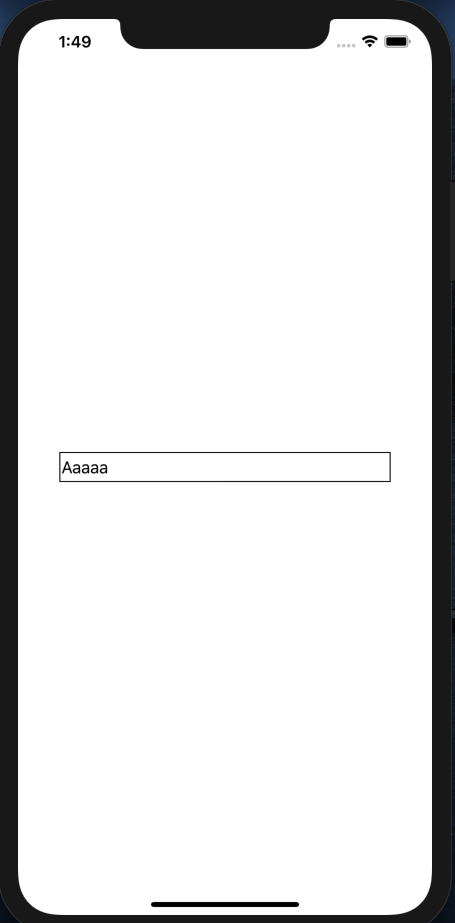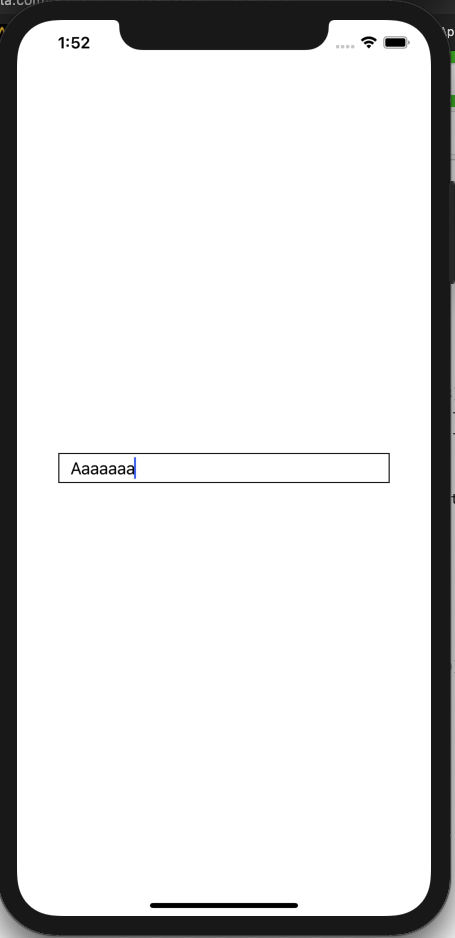# [SWIFT] Make a margin to the left of the TextField

TextField has properties called leftView and rightView, so I will place a transparent View there to create a margin. If you generate a TextField with the following code,

``````textField.borderStyle = .line
//Auto layout
textField.widthAnchor.constraint(equalTo: view.widthAnchor, multiplier: 0.8).isActive = true
textField.centerYAnchor.constraint(equalTo: view.centerYAnchor).isActive = true
textField.centerXAnchor.constraint(equalTo: view.centerXAnchor).isActive = true
``````The first letter is too close to the border on the left. So let's add the following code.

``````textField.leftViewMode = .always
textField.leftView = UIView(frame: CGRect(x: 0, y: 0, width: 10, height: 30))
``````I was able to add a margin! You can make a margin on the right side in the same way. You can also arrange buttons, so it seems that you can use it in various ways!